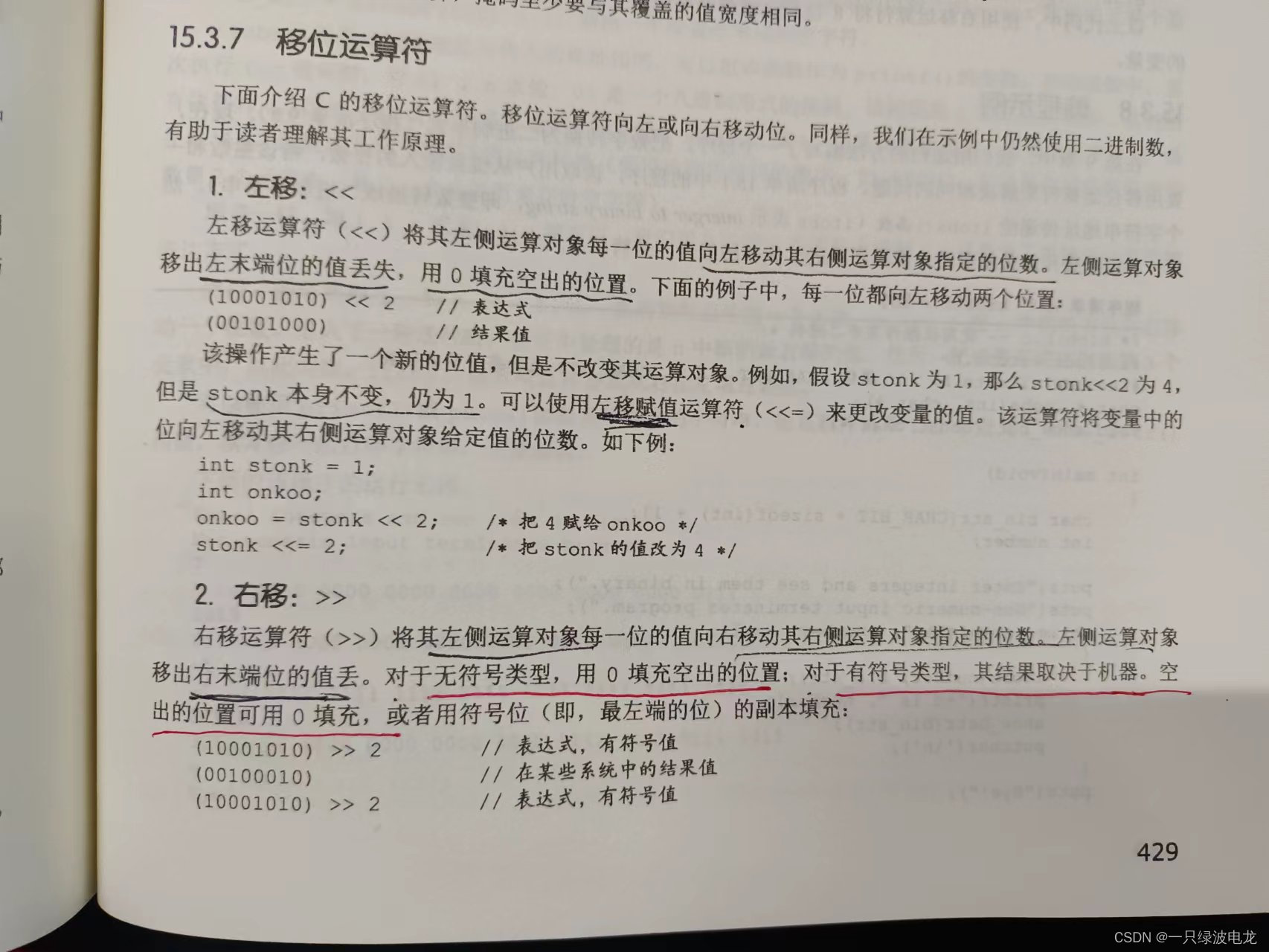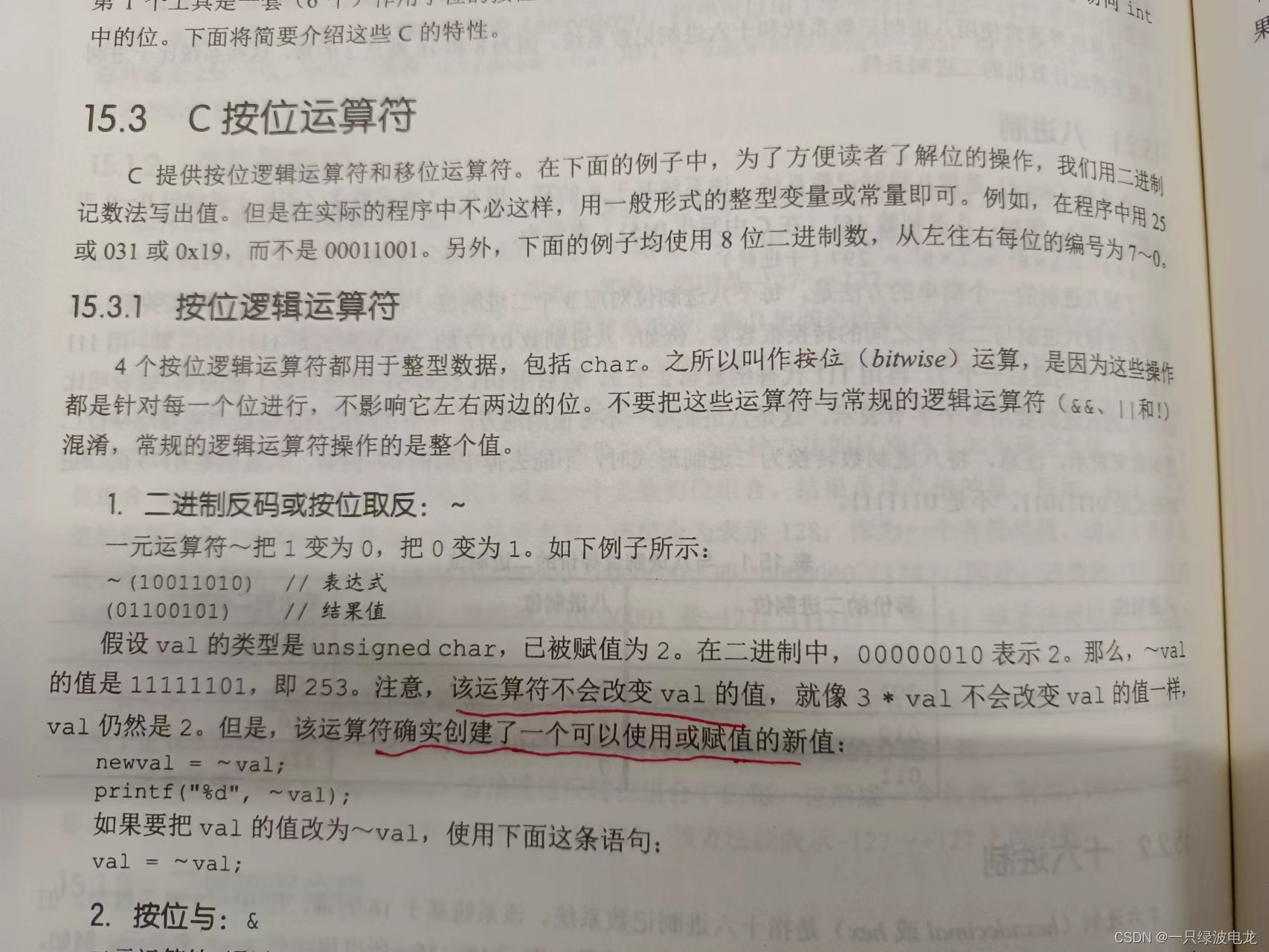# 【51单片机实现】多彩流水灯效果

1、按键 1、2、3、4 按下，使 8 个 LED 实现下面对应的模式 1、 2、        3、4，上电默认每种模式流水灯的流转时间间隔为 500ms。

1）模式1：按照L1、L2……L8的顺序，从左到右循环点亮。

2）模式2：按照L8、L7……L1的顺序，从右刀座循环点亮。

3）模式3：从两边向中间点亮(  (L1,L8)->(L2,L7)->(L3,L6)->(L4,L5)  )

4）模式4：从中间向两边点亮(  (L4,L5)->(L3,L6)->(L2,L7)->(L1,L8)  )

2、按键5按下流水灯的流转时间间隔增加100ms，超过1200ms从400ms开始，用定时器控制时间

3、代码简洁，注释简单易懂。

LED处理部分

# 实现思路：还没学过Visio画图，第一次尝试，轻喷~

## 按键部分主函数要循环判断Light_Mode变量的数值，所以写一个无参数无返回值的LED处理的函数，先根据按键返回值，给Light_Mode变量赋相应的状态值，再根据这个状态值，实现四种不同模式的点灯。

## 定时器+中断

``````void Timer0_Init(void)		//1毫秒@11.0592MHz
{
TMOD &= 0xF0;		//设置定时器模式
TMOD |= 0x01;		//设置定时器模式
TL0 = 0x66;		//设置定时初值
TH0 = 0xFC;		//设置定时初值
TF0 = 0;		//清除TF0标志
TR0 = 1;		//定时器0开始计时
ET0=1;
EA=1;
PT0=0;
}``````

``````void Timer0_Routine() interrupt 1  //定时器0的中断函数
{
static unsigned char Button; //按键扫描时间变量 10ms
static unsigned int T0Count;

TL0 = 0x66;		//设置定时初值
TH0 = 0xFC;		//设置定时初值
Button++;
T0Count++;

if(T0Count >= SpaceT){
i++;
T0Count = 0;	//软件复位
}

if(Button >= 10){
MatrixKey_Loop();
Button = 0;
}

}``````

## LED处理1111 1110

1111 1101

1111 1011

1111 0111

1110 1111

1101 1111

1011 1111

0111 1111参考书 C primer plus

……

……``````for(i=0;i<8;)	//i变量在定时器里加加，控制闪烁时间间隔
{
P2 = ~(0x01<<i);
}``````

``````for(i=0;i<8;)	//i变量在定时器里加加，控制闪烁时间间隔
{
P2 = ~(0x80>>i);

}``````

``````for(i=0;i<4;)
{
P2 = ~((0x01<<i) | (0x80>>i));//两边向中间 亮灯
}``````

``````for(i=0;i<4;)
{
P2 = ~((0x10<<i) | (0x08>>i));
}``````

# 程序源码Timer0.c

``````#include <REGX52.H>
#include "Timer0.h"

//定时器0初始化模板  1毫秒@11.0592MHz
void Timer0_Init(void)		//1毫秒@11.0592MHz
{
TMOD &= 0xF0;		//设置定时器模式
TMOD |= 0x01;		//设置定时器模式
TL0 = 0x66;		//设置定时初值
TH0 = 0xFC;		//设置定时初值
TF0 = 0;		//清除TF0标志
TR0 = 1;		//定时器0开始计时
ET0=1;
EA=1;
PT0=0;
}``````

Timer0.h

``````#ifndef __TIMER0_H__
#define __TIMER0_H__

void Timer0_Init(void);		//1毫秒@11.0592MHz

#endif``````

MatrixKeyT.c

矩阵按键模块化的代码在之前的文章有发过，需要的童鞋们可以去翻阅一下

``````#include <REGX52.H>
#include "MatrixKeyT.h"

unsigned char Key_KeyNumber;

/**
*@brief   名称：矩阵键盘扫描，获取按键键码
*@param   参数：无  （放在主函数while里 赋值给一个 代表键码的变量）
*@retval返回值：KeyNumber 0代表无按键按下，1~16代表键码
*/
unsigned char Key(void)
{
unsigned char Temp = 0;
Temp = Key_KeyNumber;	//赋值给暂存变量
Key_KeyNumber = 0;		//清零
return Temp;			//返回暂存变量
}

/**
*@brief   名称：矩阵键盘读取按键键码, 内部函数(不需要外部调用的)
*@param   参数：无
*@retval返回值：KeyNumber 0代表无按键按下，1~16代表键码
*/
unsigned char MatrixKey_GetState()
{
unsigned char KeyNumber=0;

P1=0xFF;
P1_3=0;
if(P1_7==0 && P1_3==0){KeyNumber=1;}
if(P1_6==0 && P1_3==0){KeyNumber=5;}
if(P1_5==0 && P1_3==0){KeyNumber=9;}
if(P1_4==0 && P1_3==0){KeyNumber=13;}

P1=0xFF;
P1_2=0;
if(P1_7==0 && P1_2==0){KeyNumber=2;}
if(P1_6==0 && P1_2==0){KeyNumber=6;}
if(P1_5==0 && P1_2==0){KeyNumber=10;}
if(P1_4==0 && P1_2==0){KeyNumber=14;}

P1=0xFF;
P1_1=0;
if(P1_7==0 && P1_1==0){KeyNumber=3;}
if(P1_6==0 && P1_1==0){KeyNumber=7;}
if(P1_5==0 && P1_1==0){KeyNumber=11;}
if(P1_4==0 && P1_1==0){KeyNumber=15;}

P1=0xFF;
P1_0=0;
if(P1_7==0 && P1_0==0){KeyNumber=4;}
if(P1_6==0 && P1_0==0){KeyNumber=8;}
if(P1_5==0 && P1_0==0){KeyNumber=12;}
if(P1_4==0 && P1_0==0){KeyNumber=16;}

return KeyNumber;
}

void MatrixKey_Loop(void)
{
static unsigned char NowState,LastState;
LastState = NowState;				//按键状态更新
NowState  = MatrixKey_GetState();		//获取当前按键状态
if(LastState == 1 && NowState == 0) {Key_KeyNumber=1;}
if(LastState == 2 && NowState == 0) {Key_KeyNumber=2;}
if(LastState == 3 && NowState == 0) {Key_KeyNumber=3;}
if(LastState == 4 && NowState == 0) {Key_KeyNumber=4;}
if(LastState == 5 && NowState == 0) {Key_KeyNumber=5;}
if(LastState == 6 && NowState == 0) {Key_KeyNumber=6;}
if(LastState == 7 && NowState == 0) {Key_KeyNumber=7;}
if(LastState == 8 && NowState == 0) {Key_KeyNumber=8;}
if(LastState == 9 && NowState == 0) {Key_KeyNumber=9;}
if(LastState ==10 && NowState == 0) {Key_KeyNumber=10;}
if(LastState ==11 && NowState == 0) {Key_KeyNumber=11;}
if(LastState ==12 && NowState == 0) {Key_KeyNumber=12;}
if(LastState ==13 && NowState == 0) {Key_KeyNumber=13;}
if(LastState ==14 && NowState == 0) {Key_KeyNumber=14;}
if(LastState ==15 && NowState == 0) {Key_KeyNumber=15;}
if(LastState ==16 && NowState == 0) {Key_KeyNumber=16;}
}

``````

MatrixKeyT.h

``````#ifndef __MATRIXKEYT_H__
#define __MATRIXKEYT_H__

unsigned char Key(void);
void MatrixKey_Loop(void);

#endif``````

main.c

``````#include <REGX52.H>
#include "Timer0.h"
#include "MatrixKeyT.h"

unsigned char i;//循环变量
unsigned char KeyNumer;
unsigned int  SpaceT = 500;//初值400ms
unsigned char Light_Mode;

void LED_Func();

void main()
{
Timer0_Init();

while(1)
{
KeyNumer = Key();
LED_Func();

}
}

void LED_Func()
{
if	   (KeyNumer == 1) Light_Mode=1;
else if(KeyNumer == 2) Light_Mode=2;
else if(KeyNumer == 3) Light_Mode=3;
else if(KeyNumer == 4) Light_Mode=4;
else if(KeyNumer == 5)
{
SpaceT += 100;
}
if(SpaceT>1200)
SpaceT = 400;

if(Light_Mode == 1)
{
for(i=0;i<8;)	//i变量在定时器里加加，控制闪烁时间间隔
{
P2 = ~(0x01<<i);
//				if(Light_Mode != 1)	//for循环，按键键码数值改变，但是模式没及时改变，此处有个bug
//					break;	//要小写
}
}

else if(Light_Mode == 2)
{
for(i=0;i<8;)	//i变量在定时器里加加，控制闪烁时间间隔
{
P2 = ~(0x80>>i);
//				if(Light_Mode != 2)
//					break;
}
}

else if(Light_Mode == 3)
{
for(i=0;i<4;)
{
P2 = ~((0x01<<i) | (0x80>>i));//两边向中间 亮灯
}
}

else if(Light_Mode == 4)
{
for(i=0;i<4;)
{
P2 = ~((0x10<<i) | (0x08>>i));
}
}
}

void Timer0_Routine() interrupt 1  //定时器0的中断函数
{
static unsigned char Button; //按键扫描时间变量 10ms
static unsigned int T0Count;

TL0 = 0x66;		//设置定时初值
TH0 = 0xFC;		//设置定时初值
Button++;
T0Count++;

if(T0Count >= SpaceT){
i++;
T0Count = 0;	//软件复位
}

if(Button >= 10){
MatrixKey_Loop();
Button = 0;
}

}
``````

LED流水实现部分有个BUG：因为我写的是for循环，虽然这时候按下按键，变量Light_Mode已经被重新赋值了，所以不能立刻跳出循环，执行下一个流水灯效果。之前有测试过跳出那个for循环，但是没有实现过，有兴趣的小伙伴可以尝试修改一下bug。

``````/*   void forward(void)函数说明

1111 1110<<1    1111 1100     最后一个跟着亮了，给最后一位清零，即灭，“或”0x01，变成1111 1101 ，下次赋值本数

1111 1101<<1    1111 1010    也要把最后一位清零，|0x01，变成1111 1011……
*/

void forward(void)
{
unsigned char TempOut = 0xfe,j;
for (j=0;j<8;j++)
{
P1 = TempOut;
Delay_ms(200);
TempOut = (TempOut << 1) | 0x01;

}
}``````